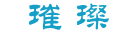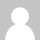﻿ HTML表单(Forms) - 璀璨's Blog

#会飞的鱼

## HTML表单(Forms)手机扫码查看

# HTML表单(Forms)

HTML表单(Form)是HTML的一个重要部分，主要用于采集和提交用户输入的信息。

`<form action="http://www.admin5.com/html/asdocs/html_tutorials/yourname.asp" method="get">`
`请输入你的姓名：`
`<input type="text" name="yourname">`
`<input type="submit" value="提交">`
`</form>`

• 表单控件(Form Controls)
• Action
• Method

## HTML表单(Form)常用控件(Controls)

HTML表单(Form)常用控件有：

 表单控件(Form Contros) 说明 input type="text" 单行文本输入框 input type="submit" 将表单(Form)里的信息提交给表单里action所指向的文件 input type="checkbox" 复选框 input type="radio" 单选框 select 下拉框 textArea 多行文本输入框 input type="password" 密码输入框(输入的文字用*表示)

### 表单控件(Form Control)：单行文本输入框(input type="text")

`<input type="text" name="yourname">`

### 表单控件(Form Control)：复选框(input type="checkbox")

`<input type="checkbox" name="fruit" value ="apple">苹果<br>`
`<input type="checkbox" name="fruit" value ="orange">桔子<br>`
`<input type="checkbox" name="fruit" value ="mango">芒果<br>`

`<input type="checkbox" name="fruit" value ="orange" checked>桔子<br>`

`<input type="radio" name="fruit" value = "Apple">苹果<br>`
`<input type="radio" name="fruit" value = "Orange">桔子<br>`
`<input type="radio" name="fruit" value = "Mango">芒果<br>`

`<input type="radio" name="fruit" value = "Orange" checked>桔子<br>`

### 表单控件(Form Control)：下拉框(select)

`<select name="fruit" >`
`  <option value="apple">苹果`
`  <option value="orange">桔子`
`  <option value="mango">芒果`
`</select>`

`<select name="fruit" multiple>`

### 表单控件(Form Control)：多行输入框(textarea)

`<textarea name="yoursuggest" cols ="50" rows = "3"></textarea>`

`<input type="password" name="yourpw">`

### 表单控件(Form Control)：提交(input type="submit")

`<input type="submit" value="提交">`

### 表单控件(Form Control)：图片提交(input type="image")

input type=image 相当于 input type=submit，不同的是，input type=image 以一个图片作为表单的提交按钮，其中 src 属性表示图片的路径。

`<input type="image" src ="images/icons/go.gif" `
`alt = "提交" NAME="imgsubmit">`

### 评论游客### 注册sitemap